Induction Examples

This document is here to give you several examples of good induction. While it discusses briefly how induction works, you are encouraged to read the Induction Handout for a more thorough discussion of induction.

Induction is really important, so the best thing to understand induction is to do it yourself. Of course, a few examples never hurt. Before we get to the induction proof, you need to understand how an inductively defined set works. We'll start by considering what induction means, leaving mathematics aside.

At its core, induction is simply the process of inclusion.  Consider some club, such as the royal order of caribou.  No one really knows what these people do, but observation tells that all they do is bowl on Wednesday nights and eat hamburgers on Sunday afternoons.  To get into this oddly elite club, a person must first know someone already in the club and meet some qualifications.  Whenever such a person is found, he undergoes an ceremony of induction (i.e., he's being included in the club).  This new person now enjoys all the rights of being a club member, and he too can bowl on Wednesday nights and eat hamburgers on Sunday afternoons.  It is also the case that once someone becomes a member of the royal order of caribou, that person is always a member.

Applying these concepts to mathematics and computer science, we find there is not much difference between the royal order of caribou and proving programs are correct.

Anatomy of an Inductively Defined Set:

 Let the set S be the smallest set such that: Base Case: Some base element(s) is in the set S Inductive Step: If an element e is in the set S, then some manipulation of e according to some rule(s) is also in the set S

Note that there are several important things about how an inductively defined set works:

• It is the smallest set that satisfies the base case and the inductive step. That means nothing else can be a part of the set. You'll see this in the next example.
• There can be more than one element in the base case.
• There can be more than one rule in the inductive step.

Examples of Inductively Defined Sets:

 Let the set of Whole Numbers (W) be the smallest set such that: Base Case: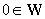Induction Step: If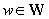then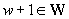Note that this defines the entire whole number set. Remember that we mentioned, it's the smallest set such that the base case and inductive step are true. You can see this when you ask whether 1.5, 2.75, or pi is in the set W? Clearly, they are not. Only 0, 1, 2, 3,... are a part of the set of whole numbers.

Note that the whole numbers are often called natural numbers.  Some people would get into arguments over whether, the natural numbers start at 0 or 1.  Using principles of inductively defined sets, why is such an argument completely pointless?

 Let the set S be the smallest set such that: Base Case: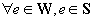Induction Step: If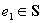and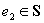then: e1 + e2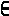S e1 - e2S e1 * e2S e1 / e2S

Notice that we have defined a small subset of the ML language. Note too that the induction step has four different rules. When we get to induction proofs later, you'll need to do the induction step of the proof for each individual induction step rule of the inductively defined set. Now one more inductively defined set before we get to induction proofs.

 Let the set L be the smallest set such that: Base Case: []L Induction Step: If xsL then x::xsL where x is a value

This is the inductive definition of a properly defined list. Notice how we've used inductively defined sets to define the set of whole numbers, the syntax of subset of ML, and the structure of lists in ML.

Anatomy of an Induction Proof:

 P(n): Some statement about n. The statement is either true or false, depending on n. Claim: Make some claim that P(n) is true for all elements of a certain set. Proof by induction on nThere are many types of induction, state which type you're using Base Case: Prove the base case of the set satisfies the property P(n). Induction Step: Let k be an element out of the set we're inducting over Assume that P(k) is true for any k (we call this The Induction Hypothesis) Prove that the rules of the inductive step in the inductively defined set preserves the property P(n).

The important thing to realize about an induction proof is that it depends on an inductively defined set (that's why we discussed this above). The property P(n) must state a property about the element n of the set you're inducting over. Now look at some examples:

Examples of Inductive Proofs:

Prove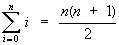P(n):Claim: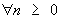, P(n) is true
Proof by induction on n

Base Case: n = 0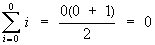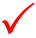Induction Step: Let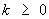Assume P(k) is true, that is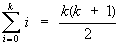[Induction Hypothesis]

Prove P(k+1) is also true: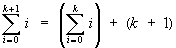[by definition of summation]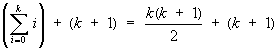[by I.H.]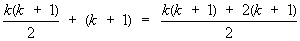[by fraction addition]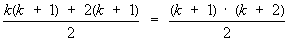[by distribution]Thus we have proven our claim is true.

QED

Notice that in this example we used the inductive definition of set of whole numbers. The base case of the induction proof uses the only element defined in the base case in the inductive definition of whole numbers. And also note that the induction step in the proof assumes P(n) is true for what we know to be in the set, and prove that the only rule of the inductive step in the inductively defined set maintains the property P(n).

Prove that every amount of postage of 12 cents or more can be formed using just 4-cent and 5-cent stamps.
P(n): "Postage of n cents can be formed using 4-cent and 5-cent stamps"
Claim: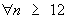, P(n) is true
Proof by strong induction on n

Base Case: n = 12, n = 13, n = 14, n = 15

• We can form postage of 12 cents using three 4-cent stamps
• We can form postage of 13 cents using two 4-cent stamps and one 5-cent stamp
• We can form postage of 14 cents using one 4-cent stamp and two 5-cent stamps
• We can form postage of 15 cents using three 5-cent stamps

Thus, P(n) is true for all elements of the base caseInduction Step: Let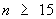Assume P(k) is true for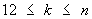, that is postage of k cents can be formed with 4-cent and 5-cent stamps. [Induction Hypothesis]

Prove that P(n + 1) is also true

To form postage of n + 1 cents, use the stamps that form postage of n - 3 cents [which exists by I.H.] together with a 4-cent stamp.Thus we have proven our claim is true.

QED

Notice two important induction techniques in this example. First we used strong induction, which allowed us to use a broader induction hypothesis. This example could also have been done with regular mathematical induction, but it would have taken many more steps in the induction step. It would be a good exercise to try and prove this without using strong induction. Second, notice how we used many elements in the base case. What is the set that we're inducting over? In this case it is a subset of the whole numbers, and it uses 12 as its base case instead of 0. Notice how we had to prove the base case for four numbers. This was necessary because we make the assumption that postage of n - 3 cents exists. If we had only proven that P(12) is true, the base case would not be sufficient to support the claim we make in the induction step.

Prove that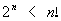for every positive integer n with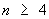P(n):Claim: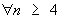, P(n) is true.
Proof by induction on n

Base Case: n = 4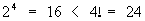Induction Step: Let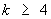Assume P(k) is true, that is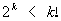[Induction Hypothesis]
Prove P(k + 1) is also true, that is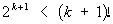[by I.H.]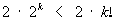[multiply both sides by 2]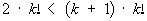[(k + 1) is larger than 2]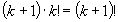[by definition of factorial]Thus we have proven that our claim is true.

QED

Notice that induction can be used to prove inequalities. Also take note that we began with the induction hypothesis and manipulated it to show what we wanted to prove. Something that you should never do is start with what you want to prove and go backwards to either show the Induction Hypothesis or some other known truth.

 Explain what is wrong with the following inductive argument that all horses are the same color. Let P(n) be the proposition that all the horses in a set of n horses are the same color. Clearly, P(1) is true. Now assume that P(n) is true, so that all the horses in any set of n horses are the same color.  Consider any n + 1 horses; number these as horses 1, 2, 3,..., n, n + 1. Now the first n of these horses all must have teh same color, and the last n of these must also have the same color. Since the set of the first n horses and the set of the last n horses overlap, all n + 1 must be the same color.  This shows that P(n + 1) is true and finishes the proof by induction. The two sets are disjoint if n + 1 = 2. In fact, the implication that P(1) implies P(2) is false.

As you can see, induction used improperly can prove ridiculous things. Often times the mistakes are subtle. It takes a good understanding of induction to use it correctly. Induction is a powerful method of proof, but in the wrong hands it can be dangerous.

Prove copy(x) = x
Given:
fun copy(l:'a list):'a list =
case l of
[]    => []
|x::xs => x::copy(xs)
P(x): copy(x) = x
Claim: P(x) is true for all properly formed lists
Proof by structural induction on L

Base Case: x = []

 copy([]) (fun copy(l) =    case l of      []    => []     |x::xs => x::copy(xs)) [] Using SM Rules: (fn l =>    case l of      []    => []     |x::xs => x::(fun copy(l) =                     case l of                       []    => []                      |x::xs => x::copy(xs)) xs)) [] Using SM Rules: case [] of   []    => []  |x::xs => x::(fun copy(l) =                  case l of                    []    => []                   |x::xs => x::copy(xs)) xs) Using SM Rules: [] Using SM Rules:Induction Step:

Assume copy(x) = x [Induction Hypothesis]
Prove that copy(v::y) = v::y

 copy(v::y) (fun copy(l) =    case l of      []    => []     |x::xs => x::copy(xs)) (v::y) Using SM Rules: (fn l =>    case l of      []    => []     |x::xs => x::(fun copy(l) =                     case l of                       []    => []                      |x::xs => x::copy(xs)) xs)) (v::y) Using SM Rules: case (v::y) of   []    => []  |x::xs => x::(fun copy(l) =                  case l of                    []    => []                   |x::xs => x::copy(xs)) xs) Using SM Rules: v::(fun copy(l) =       case l of         []    => []        |x::xs => x::copy(xs)) y) Using SM Rules: v::y [by IH]Thus we have proven that copy(x) = x for all properly formed lists.